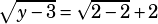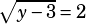Hey! I have a question from the Oct 2015 test, section 2 Q#17
Could you explain the thought process? (The parentheses are for the square root, anything in the parentheses next to the square root are meant to be included in the square root )

√(y-3) = √(x-2) +x
In the equation above, X and Y are positive integers. What is the least possible value of Y?

You’ll get the least value of y when you x is as small as possible, too. The smallest possible value of x is 2, because if x were any smaller then the square root on the right would have a negative in it. So let’s say x = 2.From there, it’s only a few more steps to the answer. Square both sides:

y – 3 = 4
y = 7R.A.R says:Mike McClenathan says: